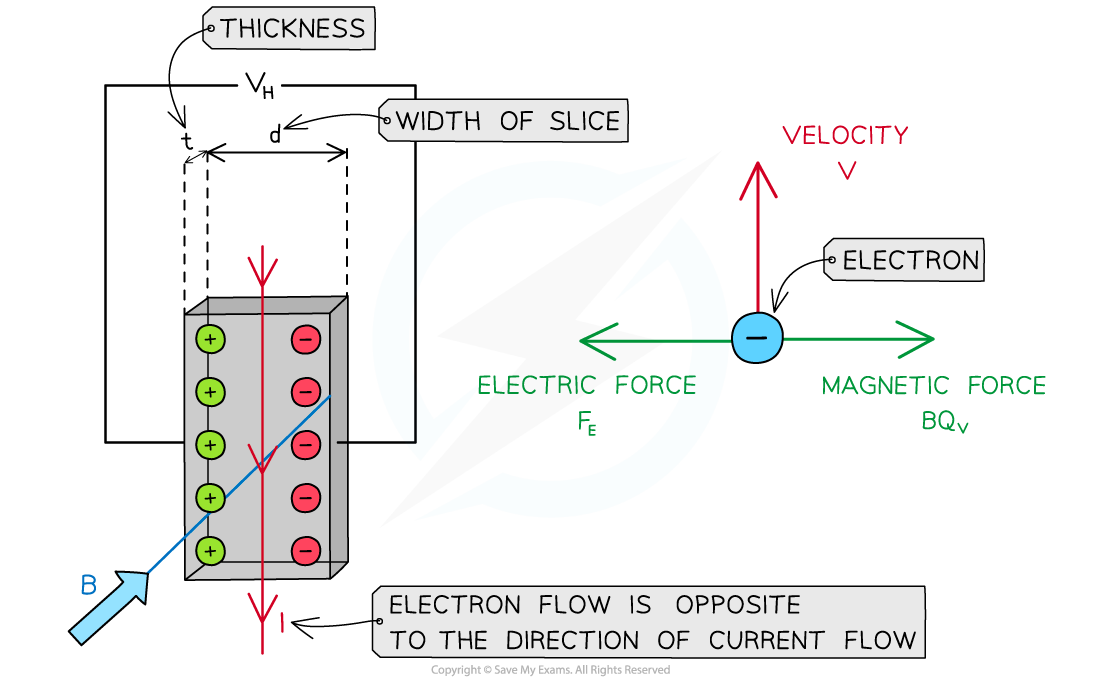# CIE A Level Physics复习笔记20.1.6 Hall Voltage

### Hall Voltage

• The Hall voltage is a product of the Hall effect
• Hall voltage is defined as:

The potential difference produced across an electrical conductor when an external magnetic field is applied perpendicular to the current through the conductor

• When an external magnetic field is applied perpendicular to the direction of current through a conductor, the electrons experience a magnetic force
• This makes them drift to one side of the conductor, where they all gather and becomes more negatively charged
• This leaves the opposite side deficient of electrons, or positively charged
• There is now a potential difference across the conductor
• This is called the Hall Voltage, VHThe positive and negative charges drift to opposite ends of the conductor producing a hall voltage when a magnetic field is applied

• An equation for the Hall voltage VH is derived from the electric and magnetic forces on the chargesThe electric and magnetic forces on the electrons are equal and opposite

• The voltage arises from the electrons accumulating on one side of the conductor slice
• As a result, an electric field is set up between the two opposite sides
• The two sides can be treated like oppositely charged parallel plates, where the electric field strength E is equal to:• Where:
• VH = Hall voltage (V)
• d = width of the conductor slice (m)
• A single electron has a drift velocity of v within the conductor. The magnetic field is into the plane of the page, therefore the electron has a magnetic force Fto the right:

FB = Bqv

• This is equal to the electric force FE to the left:

FE = qE

qE = Bqv

• Substituting E and cancelling the charge q• Recall that current I is related to the drift velocity v by the equation:

I = nAvq

• Where:
• A = cross-sectional area of the conductor (m2)
• n = number density of electrons (m-3)
• Rearranging this for v and substituting it into the equation gives:• The cross-sectional area A of the slice is the product of the width and thickness t:

A = dt

• Substituting A and rearranging for the Hall voltage VH leads to the equation:• Where:
• B = magnetic flux density (T)
• q = charge of the electron (C)
• I = current (A)
• n = number density of electrons (m-3)
• t = thickness of the conductor (m)
• This equation shows that the smaller the electron density n of a material, the larger the magnitude of the Hall voltage
• This is why a semiconducting material is often used for a Hall probe
• Note: if the electrons were placed by positive charge carriers, the negative and positive charges would still deflect in opposite directions
• This means there would be no change in the polarity (direction) of the Hall voltage

#### Exam Tip

Remember to use Fleming’s left-hand rule to obtain the direction the electrons move due to the magnetic force created by the magnetic field.